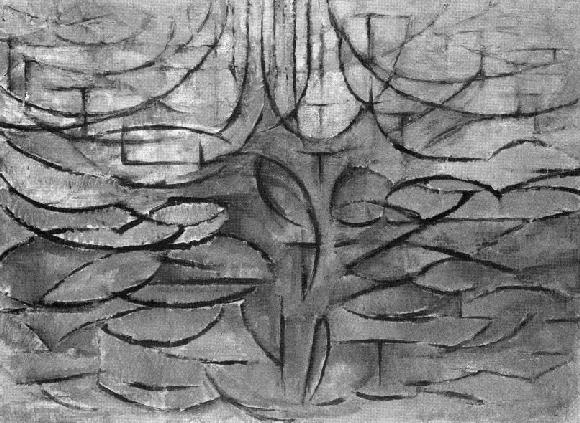Apple Tree in Blossom, Piet Mondrian (1912)

Simply Scheme: Introducing Computer Science ch 18: Trees

Simply Scheme: Introducing Computer Science 2/e Copyright (C) 1999 MIT

# TreesThe big advantage of full-featured lists over sentences is their ability to represent structure in our data by means of sublists. In this chapter we'll look at examples in which we use lists and sublists to represent two-dimensional information structures. The kinds of structures we'll consider are called trees because they resemble trees in nature: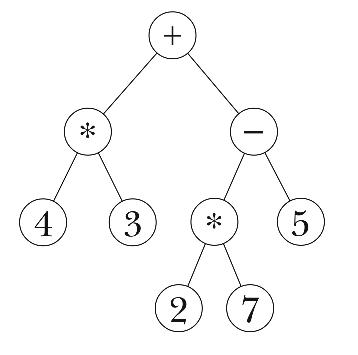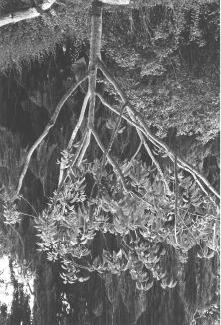The components of a tree are called nodes. At the top is the root node of the tree; in the interior of the diagram there are branch nodes; at the bottom are the leaf nodes, from which no further branches extend.

We're going to begin by considering a tree as an abstract data type, without thinking about how lists are used to represent trees. For example, we'll construct trees using a procedure named `make-node`, as if that were a Scheme primitive. About halfway through the chapter, we'll explore the relationship between trees and lists.

## Example: The World

Here is a tree that represents the world: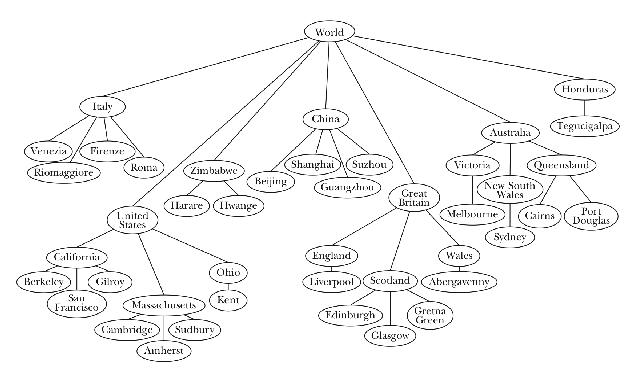Each node in the tree represents some region of the world. Consider the node labeled "Great Britain." There are two parts to this node: The obvious part is the label itself, the name "Great Britain." But the regions of the world that are included within Great Britain—that is, the nodes that are attached beneath Great Britain in the figure—are also part of this node.

We say that every node has a datum and zero or more children. For the moment, let's just say that the datum can be either a word or a sentence. The children, if any, are themselves trees. Notice that this definition is recursive—a tree is made up of trees. (What's the base case?)

This family metaphor is also part of the terminology of trees. We say that a node is the parent of another node, or that two nodes are siblings. In more advanced treatments, you even hear things like "grandparent" and "cousin," but we won't get into that.

What happens when you prune an actual tree by cutting off a branch? The cut-off part is essentially a tree in itself, with a smaller trunk and fewer branches. The metaphor isn't perfect because the cut-off part doesn't have roots, but still, we can stick the end in the ground and hope that the cut-off end will take root as a new tree.

It's the same with a country in our example; each country is a branch node of the entire world tree, but also a tree in itself. Depending on how you think about it, Great Britain can be either a component of the entire world or a collection of smaller locations. So the branch node that represents Great Britain is the root node of a subtree of the entire tree.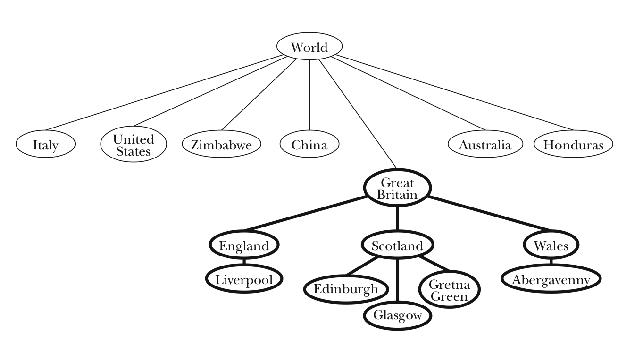What is a node? It might seem natural to think of a node as being just the information in one of the circles in the diagram—that is, to think of a node as including only its datum. In that way of thinking, each node would be separate from every other node, just as the words in a sentence are all separate elements. However, it will be more useful to think of a node as a structure that includes everything below that circle also: the datum and the children. So when we think of the node for Great Britain, we're thinking not only of the name "Great Britain," but also of everything in Great Britain. From this perspective, the root node of a tree includes the entire tree. We might as well say that the node is the tree.

The constructor for a tree is actually the constructor for one node, its root node. Our constructor for trees is therefore called `make-node`. It takes two arguments: the datum and a (possibly empty) list of children. As the following example shows, constructing what we think of as one tree requires the construction of many such nodes.

```(define world-tree                           ;; painful-to-type version
(make-node
'world
(list (make-node
'italy
(list (make-node 'venezia '())
(make-node 'riomaggiore '())
(make-node 'firenze '())
(make-node 'roma '())))
(make-node
'(united states)
(list (make-node 'california
(list (make-node 'berkeley '())
(make-node '(san francisco) '())
(make-node 'gilroy '())))
(make-node 'massachusetts
(list (make-node 'cambridge '())
(make-node 'amherst '())
(make-node 'sudbury '()))))))))
```

You'll notice that we haven't defined all of the places shown in the figure. That's because we got tired of doing all this typing; we're going to invent some abbreviations later. For now, we'll take time out to show you the selectors for trees.

```> (datum world-tree)
WORLD

> (datum (car (children world-tree)))
ITALY

> (datum (car (children (cadr (children world-tree)))))
CALIFORNIA

> (datum (car (children (car (children
BERKELEY
```

`Datum` of a tree node returns the datum of that node. `Children` of a node returns a list of the children of the node. (A list of trees is called a forest.)

Here are some abbreviations to help us construct the world tree with less typing. Unlike `make-node`, `datum`, and `children`, which are intended to work on trees in general, these abbreviations were designed with the world tree specifically in mind:

```(define (leaf datum)
(make-node datum '()))

(define (cities name-list)
(map leaf name-list))
```

With these abbreviations the world tree is somewhat easier to define:

```(define world-tree
(make-node
'world
(list (make-node
'italy
(cities '(venezia riomaggiore firenze roma)))
(make-node
'(united states)
(list (make-node
'california
(cities '(berkeley (san francisco) gilroy)))
(make-node
'massachusetts
(cities '(cambridge amherst sudbury)))
(make-node 'ohio (cities '(kent)))))
(make-node 'zimbabwe (cities '(harare hwange)))
(make-node 'china
(cities '(beijing shanghai guangzhou suzhou)))
(make-node
'(great britain)
(list
(make-node 'england (cities '(liverpool)))
(make-node 'scotland
(cities '(edinburgh glasgow (gretna green))))
(make-node 'wales (cities '(abergavenny)))))
(make-node
'australia
(list
(make-node 'victoria (cities '(melbourne)))
(make-node '(new south wales) (cities '(sydney)))
(make-node 'queensland
(cities '(cairns (port douglas))))))
(make-node 'honduras (cities '(tegucigalpa))))))
```

## How Big Is My Tree?

Now that we have the tree, how many cities are there in our world?

```(define (count-leaves tree)
(if (leaf? tree)
1
(reduce + (map count-leaves (children tree)))))

(define (leaf? node)
(null? (children node)))

> (count-leaves world-tree)
27
```

At first glance, this may seem like a simple case of recursion, with `count-leaves` calling `count-leaves`. But since what looks like a single recursive call is really a call to `map`, it is equivalent to several recursive calls, one for each child of the given tree node.

## Mutual Recursion

In Chapter 14 we wrote recursive procedures that were equivalent to using higher-order functions. Let's do the same for `count-leaves`.

```(define (count-leaves tree)
(if (leaf? tree)
1
(count-leaves-in-forest (children tree))))

(define (count-leaves-in-forest forest)
(if (null? forest)
0
(+ (count-leaves (car forest))
(count-leaves-in-forest (cdr forest)))))
```

Note that `count-leaves` calls `count-leaves-in-forest`, and `count-leaves-``in-``forest` calls `count-leaves`. This pattern is called mutual recursion.

Mutual recursion is often a useful technique for dealing with trees. In the typical recursion we've seen before this chapter, we've moved sequentially through a list or sentence, with each recursive call taking us one step to the right. In the following paragraphs we present three different models to help you think about how the shape of a tree gives rise to a mutual recursion.

In the first model, we're going to think of `count-leaves` as an initialization procedure, and `count-leaves-in-forest` as its helper procedure. Suppose we want to count the leaves of a tree. Unless the argument is a very shallow tree, this will involve counting the leaves of all of the children of that tree. What we want is a straightforward sequential recursion over the list of children. But we're given the wrong argument: the tree itself, not its list of children. So we need an initialization procedure, `count-leaves`, whose job is to extract the list of children and invoke a helper procedure, `count-leaves-in-forest`, with that list as argument.

The helper procedure follows the usual sequential list pattern: Do something to the `car` of the list, and recursively handle the `cdr` of the list. Now, what do we have to do to the `car`? In the usual sequential recursion, the `car` of the list is something simple, such as a word. What's special about trees is that here the `car` is itself a tree, just like the entire data structure we started with. Therefore, we must invoke a procedure whose domain is trees: `count-leaves`.

This model is built on two ideas. One is the idea of the domain of a function; the reason we need two procedures is that we need one that takes a tree as its argument and one that takes a list of trees as its argument. The other idea is the leap of faith; we assume that the invocation of `count-leaves` within `count-leaves-in-forest` will correctly handle each child without tracing the exact sequence of events.

The second model is easier to state but less rigorous. Because of the two-dimensional nature of trees, in order to visit every node we have to be able to move in two different directions. From a given node we have to be able to move down to its children, but from each child we must be able to move across to its next sibling.

The job of `count-leaves-in-forest` is to move from left to right through a list of children. (It does this using the more familiar kind of recursion, in which it invokes itself directly.) The downward motion happens in `count-leaves`, which moves down one level by invoking `children`. How does the program move down more than one level? At each level, `count-leaves` is invoked recursively from `count-leaves-in-forest`.

The third model is also based on the two-dimensional nature of trees. Imagine for a moment that each node in the tree has at most one child. In that case, `count-leaves` could move from the root down to the single leaf with a structure very similar to the actual procedure, but carrying out a sequential recursion:

```(define (count-leaf tree)
(if (leaf? tree)
1
(count-leaf (child tree))))
```

The trouble with this, of course, is that at each downward step there isn't a single "next" node. Instead of a single path from the root to the leaf, there are multiple paths from the root to many leaves. To make our idea of downward motion through sequential recursion work in a real tree, at each level we must "clone" `count-leaves` as many times as there are children. `Count-leaves-in-forest` is the factory that manufactures the clones. It hires one `count-leaves` little person for each child and accumulates their results.

The key point in recursion on trees is that each child of a tree is itself a perfectly good tree. This recursiveness in the nature of trees gives rise to a very recursive structure for programs that use trees. The reason we say "very" recursive is that each invocation of `count-leaves` causes not just one but several recursive invocations, one for each child, by way of `count-leaves-in-forest`.

In fact, we use the name tree recursion for any situation in which a procedure invocation results in more than one recursive call, even if there isn't an argument that's a tree. The computation of Fibonacci numbers from Chapter 13 is an example of a tree recursion with no tree. The `car`-`cdr` recursions in Chapter 17 are also tree recursions; any structured list-of-lists has a somewhat tree-like, two-dimensional character even though it doesn't use the formal mechanisms we're exploring in this chapter. The `cdr` recursion is a "horizontal" one, moving from one element to another within the same list; the `car` recursion is a "vertical" one, exploring a sublist of the given list.

## Searching for a Datum in the Tree

Procedures that explore trees aren't always as simple as `count-leaves`. We started with that example because we could write it using higher-order functions, so that you'd understand the structure of the problem before we had to take on the complexity of mutual recursion. But many tree problems don't quite fit our higher-order functions.

For example, let's write a predicate `in-tree?` that takes the name of a place and a tree as arguments and tells whether or not that place is in the tree. It is possible to make it work with `filter`:

```(define (in-tree? place tree)
(or (equal? place (datum tree))
(not (null? (filter (lambda (subtree) (in-tree? place subtree))
(children tree))))))
```

This awkward construction, however, also performs unnecessary computation. If the place we're looking for happens to be in the first child of a node, `filter` will nevertheless look in all the other children as well. We can do better by replacing the use of `filter` with a mutual recursion:

```(define (in-tree? place tree)
(or (equal? place (datum tree))
(in-forest? place (children tree))))

(define (in-forest? place forest)
(if (null? forest)
#f
(or (in-tree? place (car forest))
(in-forest? place (cdr forest)))))

> (in-tree? 'abergavenny world-tree)
#T

> (in-tree? 'abbenay world-tree)
#F

> (in-tree? 'venezia (cadr (children world-tree)))
#F
```

Although any mutual recursion is a little tricky to read, the structure of this program does fit the way we'd describe the algorithm in English. A place is in a tree if one of two conditions holds: the place is the datum at the root of the tree, or the place is (recursively) in one of the child trees of this tree. That's what `in-tree?` says. As for `in-forest?`, it says that a place is in one of a group of trees if the place is in the first tree, or if it's in one of the remaining trees.

## Locating a Datum in the Tree

Our next project is similar to the previous one, but a little more intricate. We'd like to be able to locate a city and find out all of the larger regions that enclose the city. For example, we want to say

```> (locate 'berkeley world-tree)
(WORLD (UNITED STATES) CALIFORNIA BERKELEY)
```

Instead of just getting a yes-or-no answer about whether a city is in the tree, we now want to find out where it is.

The algorithm is recursive: To look for Berkeley within the world, we need to be able to look for Berkeley within any subtree. The `world` node has several children (countries). `Locate` recursively asks each of those children to find a path to Berkeley. All but one of the children return `#f`, because they can't find Berkeley within their territory. But the `(united states)` node returns

```((UNITED STATES) CALIFORNIA BERKELEY)
```

To make a complete path, we just prepend the name of the current node, `world`, to this path. What happens when `locate` tries to look for Berkeley in Australia? Since all of Australia's children return `#f`, there is no path to Berkeley from Australia, so `locate` returns `#f`.

```(define (locate city tree)
(if (equal? city (datum tree))
(list city)
(let ((subpath (locate-in-forest city (children tree))))
(if subpath
(cons (datum tree) subpath)
#f))))

(define (locate-in-forest city forest)
(if (null? forest)
#f
(or (locate city (car forest))
(locate-in-forest city (cdr forest)))))
```

Compare the structure of `locate` with that of `in-tree?`. The helper procedures `in-forest?` and `locate-in-forest` are almost identical. The main procedures look different, because `locate` has a harder job, but both of them check for two possibilities: The city might be the datum of the argument node, or it might belong to one of the child trees.

## Representing Trees as Lists

We've done a lot with trees, but we haven't yet talked about the way Scheme stores trees internally. How do `make-node`, `datum`, and `children` work? It turns out to be very convenient to represent trees in terms of lists.

```(define (make-node datum children)
(cons datum children))

(define (datum node)
(car node))

(define (children node)
(cdr node))
```

In other words, a tree is a list whose first element is the datum and whose remaining elements are subtrees.

```> world-tree
(WORLD
(ITALY (VENEZIA) (RIOMAGGIORE) (FIRENZE) (ROMA))
((UNITED STATES)
(CALIFORNIA (BERKELEY) ((SAN FRANCISCO)) (GILROY))
(MASSACHUSETTS (CAMBRIDGE) (AMHERST) (SUDBURY))
(OHIO (KENT)))
(ZIMBABWE (HARARE) (HWANGE))
(CHINA (BEIJING) (SHANGHAI) (GUANGSZHOU) (SUZHOW))
((GREAT BRITAIN)
(ENGLAND (LIVERPOOL))
(SCOTLAND (EDINBURGH) (GLASGOW) ((GRETNA GREEN)))
(WALES (ABERGAVENNY)))
(AUSTRALIA
(VICTORIA (MELBOURNE))
((NEW SOUTH WALES) (SYDNEY))
(QUEENSLAND (CAIRNS) ((PORT DOUGLAS))))
(HONDURAS (TEGUCIGALPA)))
```

```> (car (children world-tree))
(ITALY (VENEZIA) (RIOMAGGIORE) (FIRENZE) (ROMA))
```

Ordinarily, however, we're not going to print out trees in their entirety. As in the `locate` example, we'll extract just some subset of the information and put it in a more readable form.

## Abstract Data Types

The procedures `make-node`, `datum`, and `children` define an abstract data type for trees. Using this ADT, we were able to write several useful procedures to manipulate trees before pinning down exactly how a tree is represented as a Scheme list.

Although it would be possible to refer to the parts of a node by using `car` and `cdr` directly, your programs will be more readable if you use the ADT-specific selectors and constructors. Consider this example:

```(in-tree? 'venezia (caddr world-tree))
```

What does `caddr` mean in this context? Is the `caddr` of a tree a datum? A child? A forest? Of course you could work it out by careful reasoning, but the form in which we presented this example originally was much clearer:

```(in-tree? 'venezia (cadr (children world-tree)))
```

Even better would be

```(in-tree? 'venezia (list-ref (children world-tree) 1))
```

Using the appropriate selectors and constructors is called respecting the data abstraction. Failing to use the appropriate selectors and constructors is called a data abstraction violation.

Since we wrote the selectors and constructor for trees ourselves, we could have defined them to use some different representation:

```(define (make-node datum children)
(list 'the 'node 'with 'datum datum 'and 'children children))

(define (datum node) (list-ref node 4))

(define (children node) (list-ref node 7))
```

```> (make-node 'italy (cities '(venezia riomaggiore firenze roma)))
(THE NODE WITH DATUM ITALY AND CHILDREN
((THE NODE WITH DATUM VENEZIA AND CHILDREN ())
(THE NODE WITH DATUM RIOMAGGIORE AND CHILDREN ())
(THE NODE WITH DATUM FIRENZE AND CHILDREN ())
(THE NODE WITH DATUM ROMA AND CHILDREN ())))
```

You might expect that this change in the representation would require changes to all the procedures we wrote earlier, such as `count-leaves`. But in fact, those procedures would continue to work perfectly because they don't see the representation. (They respect the data abstraction.) As long as `datum` and `children` find the right information, it doesn't matter how the trees are stored. All that matters is that the constructors and selectors have to be compatible with each other.

On the other hand, the example in this section in which we violated the data abstraction by using `caddr` to find the second child of `world-tree` would fail if we changed the representation. Many cases like this one, in which formerly working programs failed after a change in representation, led programmers to use such moralistic terms as "respecting" and "violating" data abstractions.

## An Advanced Example: Parsing Arithmetic Expressions

Consider the notation for arithmetic expressions. Scheme uses prefix notation: `(+ 3 4)`. By contrast, people who aren't Scheme programmers generally represent arithmetic computations using an infix notation, in which the function symbol goes between two arguments: 3+4.

Our goal in this section is to translate an infix arithmetic expression into a tree representing the computation. This translation process is called parsing the expression. For example, we'll turn the expression

4+3×7-5/(3+4)+6

into the treeThe point of using a tree is that it's going to be very easy to perform the computation once we have it in tree form. In the original infix form, it's hard to know what to do first, because there are precedence rules that determine an implicit grouping: Multiplication and division come before addition and subtraction; operations with the same precedence are done from left to right. Our sample expression is equivalent to

(((4 + (3 × 7)) - (5 / (3+4))) + 6)

In the tree representation, it's easy to see that the operations nearer the leaves are done first; the root node is the last operation, because it depends on the results of lower-level operations.

Our program will take as its argument an infix arithmetic expression in the form of a list:

```> (parse '(4 + 3 * 7 - 5 / (3 + 4) + 6))
```

Each element of the list must be one of three things: a number; one of the four symbols `+`, `-`, `*`, or `/`; or a sublist (such as the three-element list `(3 + 4)` in this example) satisfying the same rule. (You can imagine that we're implementing a pocket calculator. If we were implementing a computer programming language, then we'd also accept variable names as operands. But we're not bothering with that complication because it doesn't really affect the part of the problem about turning the expression into a tree.)

What makes this problem tricky is that we can't put the list elements into the tree as soon as we see them. For example, the first three elements of our sample list are `4`, `+`, and `3`. It's tempting to build a subtree of those three elements: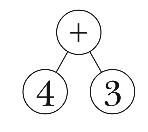But if you compare this picture with the earlier picture of the correct tree, you'll see that the second argument to this `+` invocation isn't the number `3`, but rather the subexpression `3 * 7`.

By this reasoning you might think that we have to examine the entire expression before we can start building the tree. But in fact we can sometimes build a subtree with confidence. For example, when we see the minus sign in our sample expression, we can tell that the subexpression `3 * 7` that comes before it is complete, because `*` has higher precedence than `-` does.

Here's the plan. The program will examine its argument from left to right. Since the program can't finish processing each list element right away, it has to maintain information about the elements that have been examined but not entirely processed. It's going to be easier to maintain that information in two parts: one list for still-pending operations and another for still-pending operands. Here are the first steps in parsing our sample expression; the program examines the elements of the argument, putting numbers onto the operand list and operation symbols onto the operation list: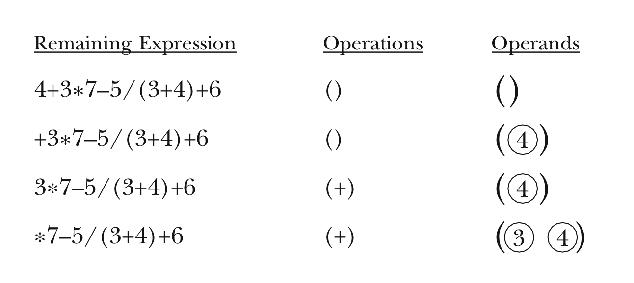At this point, the program is looking at the `*` operator in the infix expression. If this newly seen operator had lower precedence than the `+` that's already at the head of the list of operations, then it would be time to carry out the `+` operation by creating a tree with `+` at the root and the first two operands in the list as its children. Instead, since `*` has higher precedence than `+`, the program isn't ready to create a subtree but must instead add the `*` to its operation list.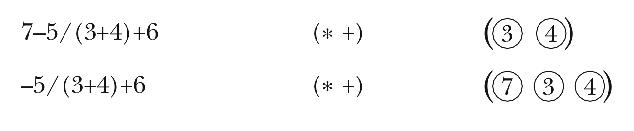This time, the newly seen `-` operation has lower precedence than the `*` at the head of the operation list. Therefore, it's time for the program to handle the `*` operator, by making a subtree containing that operator and the first two elements of the operand list. This new subtree becomes the new first element of the operand list.Because the program decided to handle the waiting `*` operator, it still hasn't moved the `-` operator from the infix expression to the operator list. Now the program must compare `-` with the `+` at the head of the list. These two operators have the same precedence. Since we want to carry out same-precedence operators from left to right, it's time to handle the `+` operator.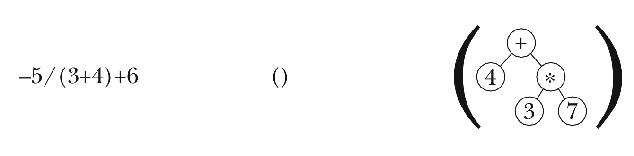Finally the program can move the `-` operator onto the operator list. The next several steps are similar to ones we've already seen.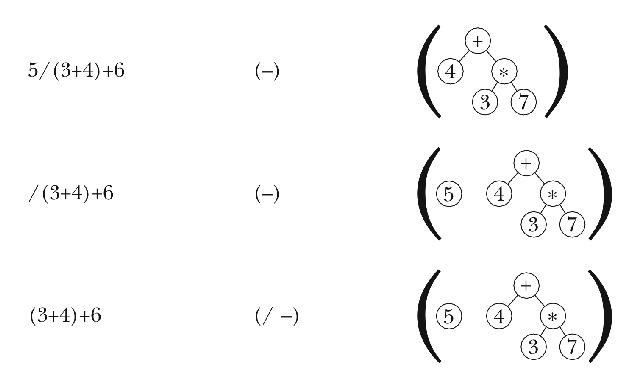This is a new situation: The first unseen element of the infix expression is neither a number nor an operator, but a sublist. We recursively `parse` this subexpression, adding the resulting tree to the operand list.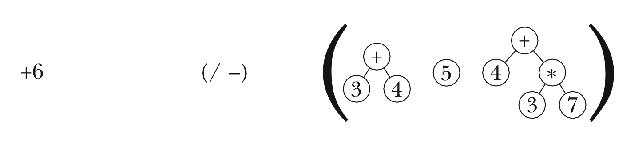Then we proceed as before, processing the `/` because it has higher precedence than the `+`, then the `-` because it has the same priority as the `+`, and so on.Once the program has examined every element of the infix expression, the operators remaining on the operator list must be handled. In this case there is only one such operator. Once the operators have all been handled, there should be one element remaining on the operand list; that element is the desired tree for the entire original expression.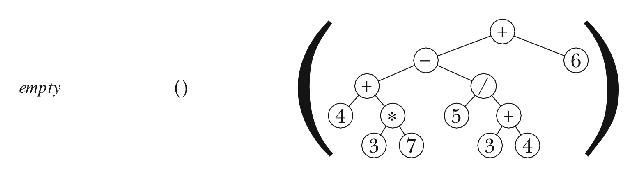The following program implements this algorithm. It works only for correctly formed infix expressions; if given an argument like `(3 + *)`, it'll give an incorrect result or a Scheme error.

```(define (parse expr)
(parse-helper expr '() '()))

(define (parse-helper expr operators operands)
(cond ((null? expr)
(if (null? operators)
(car operands)
(handle-op '() operators operands)))
((number? (car expr))
(parse-helper (cdr expr)
operators
(cons (make-node (car expr) '()) operands)))
((list? (car expr))
(parse-helper (cdr expr)
operators
(cons (parse (car expr)) operands)))
(else (if (or (null? operators)
(> (precedence (car expr))
(precedence (car operators))))
(parse-helper (cdr expr)
(cons (car expr) operators)
operands)
(handle-op expr operators operands)))))

(define (handle-op expr operators operands)
(parse-helper expr
(cdr operators)
(cons (make-node (car operators)
(cddr operands))))

(define (precedence oper)
(if (member? oper '(+ -)) 1 2))
```

We promised that after building the tree it would be easy to compute the value of the expression. Here is the program to do that:

```(define (compute tree)
(if (number? (datum tree))
(datum tree)
((function-named-by (datum tree))
(compute (car (children tree)))

(define (function-named-by oper)
(cond ((equal? oper '+) +)
((equal? oper '-) -)
((equal? oper '*) *)
((equal? oper '/) /)
(else (error "no such operator as" oper))))

> (compute (parse '(4 + 3 * 7 - 5 / (3 + 4) + 6)))
30.285714285714
```

## Pitfalls

A leaf node is a perfectly good actual argument to a tree procedure, even though the picture of a leaf node doesn't look treeish because there aren't any branches. A common mistake is to make the base case of the recursion be a node whose children are leaves, instead of a node that's a leaf itself.

The value returned by `children` is not a tree, but a forest. It's therefore not a suitable actual argument to a procedure that expects a tree.

## Exercises

18.1  What does

```((SAN FRANCISCO))
```

mean in the printout of `world-tree`? Why two sets of parentheses?

18.2  Suppose we change the definition of the tree constructor so that it uses `list` instead of `cons`:

```(define (make-node datum children)
(list datum children))
```

How do we have to change the selectors so that everything still works?

18.3  Write `depth,` a procedure that takes a tree as argument and returns the largest number of nodes connected through parent-child links. That is, a leaf node has depth 1; a tree in which all the children of the root node are leaves has depth 2. Our world tree has depth 4 (because the longest path from the root to a leaf is, for example, world, country, state, city).

18.4  Write `count-nodes`, a procedure that takes a tree as argument and returns the total number of nodes in the tree. (Earlier we counted the number of leaf nodes.)

18.5  Write `prune`, a procedure that takes a tree as argument and returns a copy of the tree, but with all the leaf nodes of the original tree removed. (If the argument to `prune` is a one-node tree, in which the root node has no children, then `prune` should return `#f` because the result of removing the root node wouldn't be a tree.)

18.6  Write a program `parse-scheme` that parses a Scheme arithmetic expression into the same kind of tree that `parse` produces for infix expressions. Assume that all procedure invocations in the Scheme expression have two arguments.

The resulting tree should be a valid argument to `compute`:

```> (compute (parse-scheme '(* (+ 4 3) 2)))
14
```

(You can solve this problem without the restriction to two-argument invocations if you rewrite `compute` so that it doesn't assume every branch node has two children.)

 Contrariwise, the tree metaphor is also part of the terminology of families.

 You probably think of trees as being short or tall. But since our trees are upside-down, the convention is to call them shallow or deep.

 Another example of a data abstraction violation is in Chapter 16. When `match` creates an empty known-values database, we didn't use a constructor. Instead, we merely used a quoted empty sentence:

```(define (match pattern sent)
(match-using-known-values pattern sent '()))
```

 Actually, as we'll see shortly, the elements of the operand list are trees, so what we put in the operand list is a one-node tree whose datum is the number.

Brian Harvey, `bh@cs.berkeley.edu`